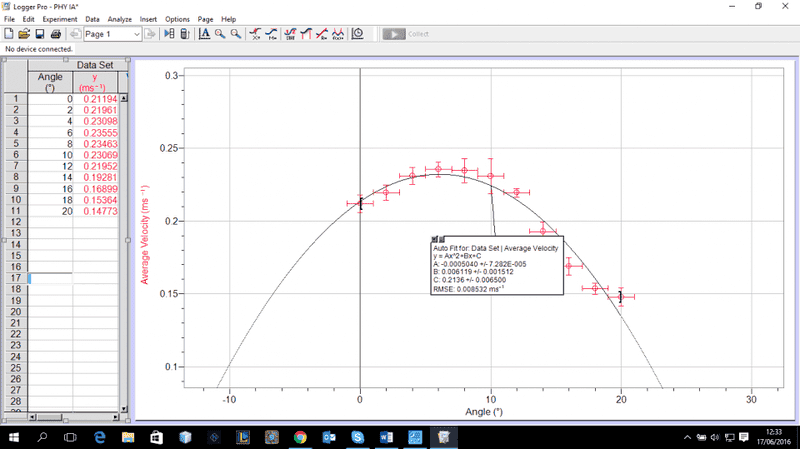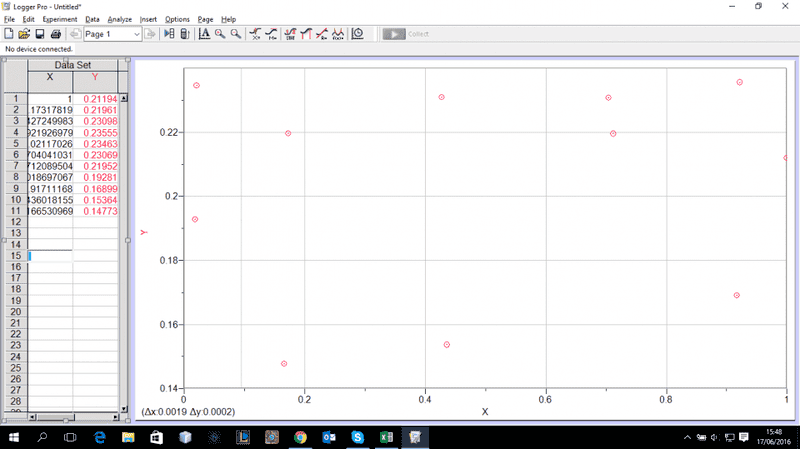# How do I linearize this graph?

• B
• Alessandro Angeletti
I'm not sure why you would want to use a different equation when the original equation works just fine.I squared the actual raw data [or coordinates if you will] for example for the first data point 0.21194 I squared that and proceeded to do that for all the other points and then plotted that however I only obtained the same shape of the curveIn summary, this person is trying to linearize a graph that has the form y = ax2 + bx + c. When they plotted y/x against 1f

#### Alessandro Angeletti

Hello!
I'm completing my IB Physics IA and after collecting my data and plotting the raw graph my graph seems to be a negative parabola.
In order to linearize it I though one had to square the x-axis data; however I simply get the same graph shape.
What am I supposed to do?
Only when I plot the following I get a straight line [y-axis data = y and x-axis data = x]:
y/x against 1/x.

Why is this? How do I linearize this graph?
Thankyou!

Could you post a graph of what the y vs x data look like?

or post the raw x and y data.

my graph seems to be a negative parabola

That sounds like it has the form
y = -xn + C

If a plot of y vs -x2 is still a parabola then perhaps n is another even number like 4 or 6 etc ?

Only when I plot the following I get a straight line [y-axis data = y and x-axis data = x]:
y/x against 1/x.

If y/x against 1/x is a straight line than y vs. x should be a straight line too. I agree with CWatters that x,y-data would be helpful.

You should also explain why you want to linearize the data. Maybe there is an entirely different solution for your problem.

Thank very much for the quick replies, I've attached my raw data graph to this reply.
I'm attempting to linearize how the heeling angle of a boat affects its velocity.
Moreover I've already attempted to raise the data to a different power [up to 10] against the other variable however this doesn't seem to work either.
The data is also on the left hand side if you would like to attempt to try something out.

Thank you very much in advance!The data seems to have a nicely behaved maximum and the data points are evenly spread along the curve. What would be the purpose of "linearising" the graph? (Or do you mean something different from what I am thinking?)

I've attached my raw data graph to this reply.
I'm attempting to linearize how the heeling angle of a boat affects its velocity.
When you attempted to linearize this by squaring the x coordinate, did you square the raw value or did you square its displacement from the midpoint of the fitted parabola?

Also, since the x-axis is angle, I would expect something that goes as cos(x) or cos2(x) more than x2.

The data seems to have a nicely behaved maximum and the data points are evenly spread along the curve. What would be the purpose of "linearising" the graph? (Or do you mean something different from what I am thinking?)
I would need to linearize this graph in order to show a proportional or linear relationship between these
When you attempted to linearize this by squaring the x coordinate, did you square the raw value or did you square its displacement from the midpoint of the fitted parabola?

I squared the actual raw data [or coordinates if you will] for example for the first data point 0.21194 I squared that and proceeded to do that for all the other points and then plotted that however I only obtained the same shape of the curve

Also, since the x-axis is angle, I would expect something that goes as cos(x) or cos2(x) more than x2.

I just tried plotting velocity against cos^2(x) and its look more like a scattered graph unfortunatelyI've not used LoggerPro but Google found..

In order to linearize it I though one had to square the x-axis data; however I simply get the same graph shape.

That doesn't work as your equations is of the form
y = ax2 + bx + c

I'm not sure how you linearize a quadratic that has a bx term.

I would need to linearize this graph in order to show a proportional or linear relationship between these

The plot clearly shows that the relationship is not linear and if you transform it into a linear graph you don't need to show the linearity anymore because it has been designed to be linear. I do not see a reason for a linearization unless you have a corresponding theoretical model.

•sophiecentaur
I squared the actual raw data [or coordinates if you will] for example for the first data point 0.21194 I squared that and proceeded to do that for all the other points and then plotted that however I only obtained the same shape of the curve
In order to get a proper result, you should square the offset of each point from the fitted center of the parabola and re-draw the graph using those results.

I'm not sure how you linearize a quadratic that has a bx term.

That would be possible with y' = 2ax + b (if the data allow for a numerical differentiation) but the question is why to do something like that.

•CWatters
I would need to linearize this graph in order to show a proportional or linear relationship between these
But the relationship clearly goes through a maximum and that, I would imagine, would be worth finding as it would correspond (?) to some optimum design arrangement. It would be relatively easy to identify this maximum. This has been mentioned earlier I think.
I can't help feeling that someone may have suggested this linearising strategy to you and you feel you need to follow it. It;s an excellent idea when dealing with some types of data. I would have said that the nature of your data is telling you that there is no linear relationship. If it was, indeed, someone else's idea, have they actually seen your data? It would not be the first time on PF that someone wants help with some task that they have been set which is not necessarily appropriate. Ask your teacher / advisor / supervisor and save yourself more angst.Here is an alternative to the quadratic equation.
I've only spot checked this from 6 to 20 degrees, 5% error. Needs refinement for less than PHIvpk (6 degrees).
Have fun!

Vpk = Peak Velocity
PHI = New Heel Angle
PHIvpk = Heel Angle at Peak Velocity
VY = Velocity at PHI

VY = Vpk x COS15.3(PHI - PHIvpk)
(Yes, that is the 15.3 power of the COS)

Here is an alternative to the quadratic equation.
I've only spot checked this from 6 to 20 degrees, 5% error. Needs refinement for less than PHIvpk (6 degrees).
Have fun!

Vpk = Peak Velocity
PHI = New Heel Angle
PHIvpk = Heel Angle at Peak Velocity
VY = Velocity at PHI

VY = Vpk x COS15.3(PHI - PHIvpk)
(Yes, that is the 15.3 power of the COS)
Thank you very much for the help however when graphing COS^15.3 one can see how some areas of the graph are undefined and as a result I cannot achieve data points for some results

some areas of the graph are undefined and as a result I cannot achieve data points for some results
Which areas?

If it is areas less than PHIvpk (6 degrees), that is the "needs refinement" area I mentioned. The following should cover that.
VY = Vpk x COS15.3(ABS(PHI - PHIvpk))

Could someone help me with a reason for doing this?
The OP has not replied to my question about the purpose of the exercise but a number of you have been looking for a solution so perhaps one of you could justify what you're doing.

The only reason I can think of is that it is vaguely satisfying to see the data appear as a straight line. Sort of confirmation that the quadratic is "correct". But in reality that's like buying a tin of blue paint and then checking inside to see if it is blue... What do you expect?

•sophiecentaur
Plot y vs (x-6.07)2.

The only reason I can think of is that it is vaguely satisfying to see the data appear as a straight line. Sort of confirmation that the quadratic is "correct". But in reality that's like buying a tin of blue paint and then checking inside to see if it is blue... What do you expect?
Absolutely. Hence my earlier comment. Linearising data serves either to 'prove' a suggested formula or to find some important coefficient from it. In this instance things seem just the wrong way round. It looks like a problem generated by someone other than the OP.